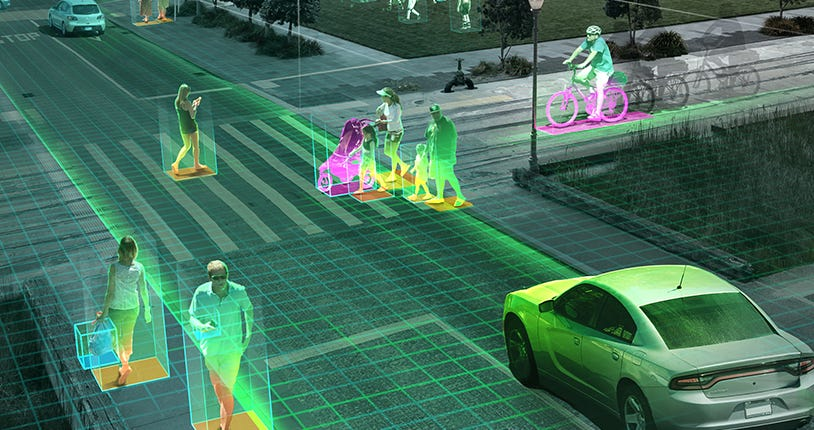# ConvCRFs for Semantic Segmentation

Source: Deep Learning on Medium## The problem of parameter learning

Parameter learning is essential to find from a large set of possible candidates the model instance that best explains the observed data and generalizes to unseen data. Despite the importance of parameter learning, current applications of random fields in computer vision sidestep many issues, making assumptions that are intuitive, but largely heuristic.

Parameter Learning in CRFs:

Whether it’s FullCRFs or CRFasRNN they both rely on hand-crafted features for pairwise (Gaussian) kernels. What actually are these features?

Let’s understand them better through an example:

An image is a typical 2D matrix with values ranging from 0 to 255. Imagine an image of entire white background with a black box placed in center. As you move from left to right you will get pixels values from 0-0-0 to 255–255–255 until you reach at the edge of box. There will be a sharp change in values and so it cannot be directly considered as a good candidate for edge detection.

It was proposed that for message passing the stated equation holds:

where kG is Gaussian kernel and Q is result on message passing

We can compute internal parameters using back propagation. However features of Gaussian kernel cannot be learned using this approach.

## The problem of inference speed

The training of time for these models are very long making any research experiments impractical.

Inference speed of CRFs:

To improve the inference speed output can be down-sampled easily. But instead this harms prediction capabilities. Hence, no significant progress was made since the introduction of FullCRFs.

# Convolutional CRFs

Do we have a solution? Yes, to enhance the FullCRFs with ConvCRFs by a conditional independence assumption. This assumption can be considered valid given that CNNs are based on local feature processing.

To make it simple, let’s take an example:

Notations:

I : Input Image

n : Total pixels

K: Classes for segmentation

i : ith Pixel

For an input image I, the pairwise potential is given by the function :

It accounts for joint distribution of pixels i,j and allows us to model interactions between pixels, such as pixels with similar colors are likely the same class. According to the locality assumption, it implies that this pairwise potential is zero for all pixels whose distance exceeds filter size.

Manhattan distance d holds d(i,j) > k(filter size)

This lead to significant reduce in complexity also making valid assumptions powerhouse of machine learning modelling.

Efficient message passing in ConvCRFs:

Without dwelling into the jargon of message passing let’s see what all changes are made for efficient message passing in ConvCRFs.

1. The operation is quite similar to standard 2d-convolutions of CNNs. However here, both spatial dimensions x and y are used as filter values.

2. Elimination of permutohedral lattice approximation and Gaussian based filtering algorithms.

3. Perform im2col(image blocks into columns) and batched dot-product over the channel dimension.

## Experimental Evaluation

The methods are evaluated on PASCAL VOC image dataset. Out of the 10852 images 200 are used for fine tuning CRF parameters and remaining for training unary CNN.Results are tested on 1464 images in validation set.

A simple FCN is added on top of the ResNet to decode the CNN features and obtain valid segmentation predictions.The CNN is trained for 200 epochs using a batch size of 16 and the adam optimizer. In addition the image colors are jittered using random brightness, random contrast, random saturation and random hue. The trained model achieves validation mIoU of 71.23% and a train mIoU of 91.84%.

Testing ConvCRFS

ConvCRFs are tested on synthetic data generated from PASCAL VOC dataset which can be visualized in figure 1.

FullCRFs are compared with ConvCRFs with same hand-crafted Gaussian features. Note that this gives FullCRFs a natural advantage.

But the results show that ConvCRFs outperform FullCRFs and are structurally superior. ConvCRFs clearly provide higher quality output.